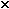The half-life of 234U, uranium-234, is 2.52105 yr. If 98% of the uranium in the original sample is present, what length of time (to the nearest thousand years) has elapsed?

m(t)=m₀e^-kt where m is the mass after t years, m₀ is the initial mass and k is a constant. When m is m₀/2 then t is the half-life=2.52×10⁵ years.

0.5=e^(-kt) where t=2.52×10⁵. So -ln(2)=-2.52×10⁵k, k=ln(2)/2.52×10⁵​=2.75×10⁻⁶.

If m=0.98m₀, 0.98=e^-2.75×10⁻⁶t, ln(0.98)=-2.75×10⁻⁶t, so t=ln(0.98)/-2.75×10⁻⁶=-0.0202/-2.75×10⁻⁶=7345 years approx.

ago by Top Rated User (735k points)